libMesh::DifferentiablePhysics Class Referenceabstract

#include <diff_physics.h>

Inheritance diagram for libMesh::DifferentiablePhysics: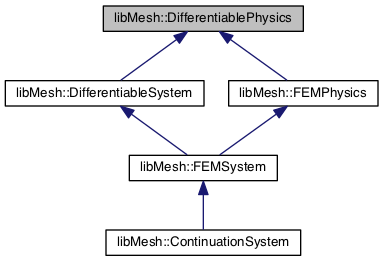[legend]

## Public Member Functions

DifferentiablePhysics ()

virtual ~DifferentiablePhysics ()

virtual std::unique_ptr< DifferentiablePhysicsclone_physics ()=0

virtual void clear_physics ()

virtual void init_physics (const System &sys)

virtual bool element_time_derivative (bool request_jacobian, DiffContext &)

virtual bool element_constraint (bool request_jacobian, DiffContext &)

virtual bool side_time_derivative (bool request_jacobian, DiffContext &)

virtual bool side_constraint (bool request_jacobian, DiffContext &)

virtual bool nonlocal_time_derivative (bool request_jacobian, DiffContext &)

virtual bool nonlocal_constraint (bool request_jacobian, DiffContext &)

virtual void time_evolving (unsigned int var)

virtual void time_evolving (unsigned int var, unsigned int order)

bool is_time_evolving (unsigned int var) const

virtual bool eulerian_residual (bool request_jacobian, DiffContext &)

virtual bool mass_residual (bool request_jacobian, DiffContext &)

virtual bool side_mass_residual (bool request_jacobian, DiffContext &)

virtual bool nonlocal_mass_residual (bool request_jacobian, DiffContext &c)

virtual bool damping_residual (bool request_jacobian, DiffContext &)

virtual bool side_damping_residual (bool request_jacobian, DiffContext &)

virtual bool nonlocal_damping_residual (bool request_jacobian, DiffContext &)

virtual void init_context (DiffContext &)

virtual void set_mesh_system (System *sys)

const Systemget_mesh_system () const

Systemget_mesh_system ()

virtual void set_mesh_x_var (unsigned int var)

unsigned int get_mesh_x_var () const

virtual void set_mesh_y_var (unsigned int var)

unsigned int get_mesh_y_var () const

virtual void set_mesh_z_var (unsigned int var)

unsigned int get_mesh_z_var () const

bool _eulerian_time_deriv (bool request_jacobian, DiffContext &)

bool have_first_order_vars () const

const std::set< unsigned int > & get_first_order_vars () const

bool is_first_order_var (unsigned int var) const

bool have_second_order_vars () const

const std::set< unsigned int > & get_second_order_vars () const

bool is_second_order_var (unsigned int var) const

## Public Attributes

bool compute_internal_sides

## Protected Attributes

System_mesh_sys

unsigned int _mesh_x_var

unsigned int _mesh_y_var

unsigned int _mesh_z_var

std::vector< unsigned int > _time_evolving

std::set< unsigned int > _first_order_vars

std::set< unsigned int > _second_order_vars

std::map< unsigned int, unsigned int > _second_order_dot_vars

## Detailed Description

This class provides a specific system class. It aims to generalize any system, linear or nonlinear, which provides both a residual and a Jacobian. For first order (in time) systems, the (nonlinear) residual computed at each time step is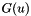for unsteady TimeSolver and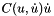for steady TimeSolver.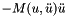is computed by element/side_time_derivative,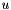is computed using element/side_constraint, and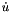is computed using the mass_residual methods.

For second order (in time) systems, the (nonlinear) residual computed at each time step is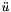for unsteady TimeSolver andfor steady TimeSolver.is computed by element/side_time_derivative,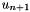is computed using element/side_constraint,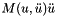is computed using the damping_residual methods and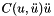is computed using the mass_residual methods. This is the sign convention used by the default implementation; if the method is overridden, the user can choose any self-consistent sign convention they wish.

FEMContext provides methods for querying values of the solution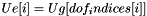, its "rate"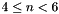and its "acceleration"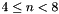. Furthermore, derivatives of each of these w.r.t the nonlinear iteration unknown (e.g. in EulerSolver, the solution at the next time step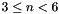) are provided through DiffContext::get_elem_solution_derivative(), DiffContext::get_elem_solution_rate_derivative(), and DiffContext::get_elem_solution_accel_derivative(). The should be incorporated into the Jacobian evaluations, if the Jacobian is being provided.

Date
2006

Definition at line 75 of file diff_physics.h.

## ◆ DifferentiablePhysics()

 libMesh::DifferentiablePhysics::DifferentiablePhysics ( )
inline

Constructor. Optionally initializes required data structures.

Definition at line 83 of file diff_physics.h.

83  :
84  compute_internal_sides (false),
85  _mesh_sys (nullptr),
89  {}
const unsigned int invalid_uint
Definition: libmesh.h:245

## ◆ ~DifferentiablePhysics()

 virtual libMesh::DifferentiablePhysics::~DifferentiablePhysics ( )
virtual

Destructor.

## ◆ _eulerian_time_deriv()

 bool libMesh::DifferentiablePhysics::_eulerian_time_deriv ( bool request_jacobian, DiffContext & )

This method simply combines element_time_derivative() and eulerian_residual(), which makes its address useful as a pointer-to-member-function when refactoring.

## ◆ clear_physics()

 virtual void libMesh::DifferentiablePhysics::clear_physics ( )
virtual

Clear any data structures associated with the physics.

Referenced by libMesh::DifferentiableSystem::clear().

## ◆ clone_physics()

 virtual std::unique_ptr libMesh::DifferentiablePhysics::clone_physics ( )
pure virtual

Copy of this object. User should override to copy any needed state.

Implemented in libMesh::DifferentiableSystem.

Referenced by libMesh::DifferentiableSystem::attach_physics().

## ◆ damping_residual()

 virtual bool libMesh::DifferentiablePhysics::damping_residual ( bool request_jacobian, DiffContext & )
inlinevirtual

Subtracts a damping vector contribution on elem from elem_residual. This method is not used in first-order-in-time problems. For second-order-in-time problems, this is the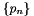term. This method is only called for UnsteadySolver-based TimeSolvers.

If this method receives request_jacobian = true, then it should compute elem_jacobian and return true if possible. If elem_jacobian has not been computed then the method should return false.

If the problem has no damping, the default "do-nothing" is correct. Otherwise, this must be reimplemented.

Definition at line 374 of file diff_physics.h.

375  {
376  return request_jacobian;
377  }

## ◆ element_constraint()

 virtual bool libMesh::DifferentiablePhysics::element_constraint ( bool request_jacobian, DiffContext & )
inlinevirtual

Adds the constraint contribution on elem to elem_residual. If this method receives request_jacobian = true, then it should compute elem_jacobian and return true if possible. If elem_jacobian has not been computed then the method should return false.

Users may need to reimplement this for their particular PDE.

To implement the constraint 0 = G(u), the user should examine u = elem_solution and add (G(u), phi_i) to elem_residual in elem_constraint().

Definition at line 142 of file diff_physics.h.

143  {
144  return request_jacobian;
145  }

## ◆ element_time_derivative()

 virtual bool libMesh::DifferentiablePhysics::element_time_derivative ( bool request_jacobian, DiffContext & )
inlinevirtual

Adds the time derivative contribution on elem to elem_residual. If this method receives request_jacobian = true, then it should compute elem_jacobian and return true if possible. If elem_jacobian has not been computed then the method should return false.

Users need to reimplement this for their particular PDE.

To implement the physics model du/dt = F(u), the user should examine u = elem_solution and add (F(u), phi_i) to elem_residual in elem_time_derivative().

Definition at line 124 of file diff_physics.h.

125  {
126  return request_jacobian;
127  }

## ◆ eulerian_residual()

 virtual bool libMesh::DifferentiablePhysics::eulerian_residual ( bool request_jacobian, DiffContext & )
inlinevirtual

Adds a pseudo-convection contribution on elem to elem_residual, if the nodes of elem are being translated by a moving mesh.

The library provides a basic implementation in FEMPhysics::eulerian_residual()

Reimplemented in libMesh::FEMPhysics.

Definition at line 294 of file diff_physics.h.

295  {
296  return request_jacobian;
297  }

## ◆ get_first_order_vars()

 const std::set& libMesh::DifferentiablePhysics::get_first_order_vars ( ) const
inline
Returns
The set of first order in time variable indices. May be empty.

Definition at line 517 of file diff_physics.h.

References _first_order_vars.

518  { return _first_order_vars; }
std::set< unsigned int > _first_order_vars
Definition: diff_physics.h:559

## ◆ get_mesh_system() [1/2]

 const System * libMesh::DifferentiablePhysics::get_mesh_system ( ) const
inline
Returns
A const reference to the system with variables corresponding to mesh nodal coordinates, or nullptr if the mesh is fixed. Useful for ALE calculations.

Definition at line 624 of file diff_physics.h.

References _mesh_sys.

Referenced by libMesh::FEMSystem::build_context().

625 {
626  return _mesh_sys;
627 }

## ◆ get_mesh_system() [2/2]

 System * libMesh::DifferentiablePhysics::get_mesh_system ( )
inline
Returns
A reference to the system with variables corresponding to mesh nodal coordinates, or nullptr if the mesh is fixed.

Definition at line 630 of file diff_physics.h.

References _mesh_sys.

631 {
632  return _mesh_sys;
633 }

## ◆ get_mesh_x_var()

 unsigned int libMesh::DifferentiablePhysics::get_mesh_x_var ( ) const
inline
Returns
The variable number corresponding to the mesh x coordinate. Useful for ALE calculations.

Definition at line 636 of file diff_physics.h.

References _mesh_x_var.

Referenced by libMesh::FEMSystem::build_context().

637 {
638  return _mesh_x_var;
639 }

## ◆ get_mesh_y_var()

 unsigned int libMesh::DifferentiablePhysics::get_mesh_y_var ( ) const
inline
Returns
The variable number corresponding to the mesh y coordinate. Useful for ALE calculations.

Definition at line 642 of file diff_physics.h.

References _mesh_y_var.

Referenced by libMesh::FEMSystem::build_context().

643 {
644  return _mesh_y_var;
645 }

## ◆ get_mesh_z_var()

 unsigned int libMesh::DifferentiablePhysics::get_mesh_z_var ( ) const
inline
Returns
The variable number corresponding to the mesh z coordinate. Useful for ALE calculations.

Definition at line 648 of file diff_physics.h.

References _mesh_z_var.

Referenced by libMesh::FEMSystem::build_context().

649 {
650  return _mesh_z_var;
651 }

## ◆ get_second_order_vars()

 const std::set& libMesh::DifferentiablePhysics::get_second_order_vars ( ) const
inline
Returns
The set of second order in time variable indices. May be empty.

Definition at line 530 of file diff_physics.h.

References _second_order_vars.

531  { return _second_order_vars; }
std::set< unsigned int > _second_order_vars
Definition: diff_physics.h:564

## ◆ have_first_order_vars()

 bool libMesh::DifferentiablePhysics::have_first_order_vars ( ) const
inline

Definition at line 511 of file diff_physics.h.

References _first_order_vars.

512  { return !_first_order_vars.empty(); }
std::set< unsigned int > _first_order_vars
Definition: diff_physics.h:559

## ◆ have_second_order_vars()

 bool libMesh::DifferentiablePhysics::have_second_order_vars ( ) const
inline

Definition at line 524 of file diff_physics.h.

References _second_order_vars.

525  { return !_second_order_vars.empty(); }
std::set< unsigned int > _second_order_vars
Definition: diff_physics.h:564

## ◆ init_context()

 virtual void libMesh::DifferentiablePhysics::init_context ( DiffContext & )
inlinevirtual

Reimplemented in libMesh::FEMSystem.

Definition at line 417 of file diff_physics.h.

417 {}

## ◆ init_physics()

 virtual void libMesh::DifferentiablePhysics::init_physics ( const System & sys )
virtual

Initialize any data structures associated with the physics.

## ◆ is_first_order_var()

 bool libMesh::DifferentiablePhysics::is_first_order_var ( unsigned int var ) const
inline

Definition at line 520 of file diff_physics.h.

References _first_order_vars.

521  { return _first_order_vars.find(var) != _first_order_vars.end(); }
std::set< unsigned int > _first_order_vars
Definition: diff_physics.h:559

## ◆ is_second_order_var()

 bool libMesh::DifferentiablePhysics::is_second_order_var ( unsigned int var ) const
inline

Definition at line 533 of file diff_physics.h.

References _second_order_vars.

534  { return _second_order_vars.find(var) != _second_order_vars.end(); }
std::set< unsigned int > _second_order_vars
Definition: diff_physics.h:564

## ◆ is_time_evolving()

 bool libMesh::DifferentiablePhysics::is_time_evolving ( unsigned int var ) const
inline
Returns
true iff variable var is evolving with respect to time. In general, the user's init() function should have set time_evolving() for any variables which behave like du/dt = F(u), and should not call time_evolving() for any variables which behave like 0 = G(u).

Definition at line 277 of file diff_physics.h.

References _time_evolving.

Referenced by libMesh::FEMSystem::init_context().

278  {
279  libmesh_assert_less(var,_time_evolving.size());
280  libmesh_assert( _time_evolving[var] == 0 ||
281  _time_evolving[var] == 1 ||
282  _time_evolving[var] == 2 );
283  return _time_evolving[var];
284  }
std::vector< unsigned int > _time_evolving
Definition: diff_physics.h:554

## ◆ mass_residual()

 virtual bool libMesh::DifferentiablePhysics::mass_residual ( bool request_jacobian, DiffContext & )
inlinevirtual

Subtracts a mass vector contribution on elem from elem_residual. For first-order-in-time problems, this is theterm. For second-order-in-time problems, this is the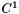term. This method is only called for UnsteadySolver-based TimeSolvers.

If this method receives request_jacobian = true, then it should compute elem_jacobian and return true if possible. If elem_jacobian has not been computed then the method should return false.

Many first-order-in-time problems can use the reimplementation in FEMPhysics::mass_residual which subtracts (du/dt,v) for each transient variable u; users with more complicated transient problems or second-order-in-time problems will need to reimplement this themselves.

Reimplemented in libMesh::FEMPhysics.

Definition at line 318 of file diff_physics.h.

319  {
320  return request_jacobian;
321  }

## ◆ nonlocal_constraint()

 virtual bool libMesh::DifferentiablePhysics::nonlocal_constraint ( bool request_jacobian, DiffContext & )
inlinevirtual

Adds any nonlocal constraint contributions (e.g. some components of constraints in scalar variable equations) to elem_residual

If this method receives request_jacobian = true, then it should also modify elem_jacobian and return true if possible. If the Jacobian changes have not been computed then the method should return false.

Users may need to reimplement this for PDEs on systems to which SCALAR variables with non-transient equations have been added.

Definition at line 227 of file diff_physics.h.

228  {
229  return request_jacobian;
230  }

## ◆ nonlocal_damping_residual()

 virtual bool libMesh::DifferentiablePhysics::nonlocal_damping_residual ( bool request_jacobian, DiffContext & )
inlinevirtual

Subtracts any nonlocal damping vector contributions (e.g. any first time derivative coefficients in scalar variable equations) from elem_residual

If this method receives request_jacobian = true, then it should also modify elem_jacobian and return true if possible. If the Jacobian changes have not been computed then the method should return false.

Definition at line 406 of file diff_physics.h.

407  {
408  return request_jacobian;
409  }

## ◆ nonlocal_mass_residual()

 virtual bool libMesh::DifferentiablePhysics::nonlocal_mass_residual ( bool request_jacobian, DiffContext & c )
virtual

Subtracts any nonlocal mass vector contributions (e.g. any time derivative coefficients in scalar variable equations) from elem_residual

If this method receives request_jacobian = true, then it should also modify elem_jacobian and return true if possible. If the Jacobian changes have not been computed then the method should return false.

Many problems can use the reimplementation in FEMPhysics::mass_residual which subtracts (du/dt,v) for each transient scalar variable u; users with more complicated transient scalar variable equations will need to reimplement this themselves.

## ◆ nonlocal_time_derivative()

 virtual bool libMesh::DifferentiablePhysics::nonlocal_time_derivative ( bool request_jacobian, DiffContext & )
inlinevirtual

Adds any nonlocal time derivative contributions (e.g. some components of time derivatives in scalar variable equations) to elem_residual

If this method receives request_jacobian = true, then it should also modify elem_jacobian and return true if possible. If the Jacobian changes have not been computed then the method should return false.

Users may need to reimplement this for PDEs on systems to which SCALAR variables have been added.

Definition at line 209 of file diff_physics.h.

210  {
211  return request_jacobian;
212  }

## ◆ set_mesh_system()

 void libMesh::DifferentiablePhysics::set_mesh_system ( System * sys )
inlinevirtual

Tells the DifferentiablePhysics that system sys contains the isoparametric Lagrangian variables which correspond to the coordinates of mesh nodes, in problems where the mesh itself is expected to move in time.

The system with mesh coordinate data (which may be this system itself, for fully coupled moving mesh problems) is currently assumed to have new (end of time step) mesh coordinates stored in solution, old (beginning of time step) mesh coordinates stored in _old_nonlinear_solution, and constant velocity motion during each time step.

Activating this function ensures that local (but not neighbor!) element geometry is correctly repositioned when evaluating element residuals.

Currently sys must be *this for a tightly coupled moving mesh problem or nullptr to stop mesh movement; loosely coupled moving mesh problems are not implemented.

This code is experimental. "Trust but verify, and not in that order"

Definition at line 580 of file diff_physics.h.

References _mesh_sys.

581 {
582  // For now we assume that we're doing fully coupled mesh motion
583  // if (sys && sys != this)
584  // libmesh_not_implemented();
585
586  // For the foreseeable future we'll assume that we keep these
587  // Systems in the same EquationSystems
588  // libmesh_assert_equal_to (&this->get_equation_systems(),
589  // &sys->get_equation_systems());
590
591  // And for the immediate future this code may not even work
592  libmesh_experimental();
593
594  _mesh_sys = sys;
595 }

## ◆ set_mesh_x_var()

 void libMesh::DifferentiablePhysics::set_mesh_x_var ( unsigned int var )
inlinevirtual

Tells the DifferentiablePhysics that variable var from the mesh system should be used to update the x coordinate of mesh nodes, in problems where the mesh itself is expected to move in time.

The system with mesh coordinate data (which may be this system itself, for fully coupled moving mesh problems) is currently assumed to have new (end of time step) mesh coordinates stored in solution, old (beginning of time step) mesh coordinates stored in _old_nonlinear_solution, and constant velocity motion during each time step.

Activating this function ensures that local (but not neighbor!) element geometry is correctly repositioned when evaluating element residuals.

Definition at line 600 of file diff_physics.h.

References _mesh_x_var.

601 {
602  _mesh_x_var = var;
603 }

## ◆ set_mesh_y_var()

 void libMesh::DifferentiablePhysics::set_mesh_y_var ( unsigned int var )
inlinevirtual

Tells the DifferentiablePhysics that variable var from the mesh system should be used to update the y coordinate of mesh nodes.

Definition at line 608 of file diff_physics.h.

References _mesh_y_var.

609 {
610  _mesh_y_var = var;
611 }

## ◆ set_mesh_z_var()

 void libMesh::DifferentiablePhysics::set_mesh_z_var ( unsigned int var )
inlinevirtual

Tells the DifferentiablePhysics that variable var from the mesh system should be used to update the z coordinate of mesh nodes.

Definition at line 616 of file diff_physics.h.

References _mesh_z_var.

617 {
618  _mesh_z_var = var;
619 }

## ◆ side_constraint()

 virtual bool libMesh::DifferentiablePhysics::side_constraint ( bool request_jacobian, DiffContext & )
inlinevirtual

Adds the constraint contribution on side of elem to elem_residual. If this method receives request_jacobian = true, then it should compute elem_jacobian and return true if possible. If elem_jacobian has not been computed then the method should return false.

Users may need to reimplement this for their particular PDE depending on the boundary conditions.

To implement a weak form of the constraint 0 = G(u), the user should examine u = elem_solution and add (G(u), phi_i) boundary integral contributions to elem_residual in side_constraint().

Definition at line 191 of file diff_physics.h.

192  {
193  return request_jacobian;
194  }

## ◆ side_damping_residual()

 virtual bool libMesh::DifferentiablePhysics::side_damping_residual ( bool request_jacobian, DiffContext & )
inlinevirtual

Subtracts a damping vector contribution on side of elem from elem_residual. If this method receives request_jacobian = true, then it should compute elem_jacobian and return true if possible. If elem_jacobian has not been computed then the method should return false.

For most problems, the default implementation of "do nothing" is correct; users with boundary conditions including first time derivatives may need to reimplement this themselves.

Definition at line 391 of file diff_physics.h.

392  {
393  return request_jacobian;
394  }

## ◆ side_mass_residual()

 virtual bool libMesh::DifferentiablePhysics::side_mass_residual ( bool request_jacobian, DiffContext & )
inlinevirtual

Subtracts a mass vector contribution on side of elem from elem_residual. If this method receives request_jacobian = true, then it should compute elem_jacobian and return true if possible. If elem_jacobian has not been computed then the method should return false.

For most problems, the default implementation of "do nothing" is correct; users with boundary conditions including time derivatives may need to reimplement this themselves.

Definition at line 335 of file diff_physics.h.

336  {
337  return request_jacobian;
338  }

## ◆ side_time_derivative()

 virtual bool libMesh::DifferentiablePhysics::side_time_derivative ( bool request_jacobian, DiffContext & )
inlinevirtual

Adds the time derivative contribution on side of elem to elem_residual. If this method receives request_jacobian = true, then it should compute elem_jacobian and return true if possible. If elem_jacobian has not been computed then the method should return false.

Users may need to reimplement this for their particular PDE depending on the boundary conditions.

To implement a weak form of the source term du/dt = F(u) on sides, such as might arise in a flux boundary condition, the user should examine u = elem_solution and add (F(u), phi_i) boundary integral contributions to elem_residual in side_constraint().

Definition at line 171 of file diff_physics.h.

172  {
173  return request_jacobian;
174  }

## ◆ time_evolving() [1/2]

 virtual void libMesh::DifferentiablePhysics::time_evolving ( unsigned int var )
inlinevirtual

Tells the DiffSystem that variable var is evolving with respect to time. In general, the user's init() function should call time_evolving() for any variables which behave like du/dt = F(u), and should not call time_evolving() for any variables which behave like 0 = G(u).

Most derived systems will not have to reimplement this function; however any system which reimplements mass_residual() may have to reimplement time_evolving() to prepare data structures.

Deprecated:
Instead, use the time_evolving override and specify the order-in-time of the variable, either 1 or 2. This method assumes the variable is first order for backward compatibility.

Definition at line 249 of file diff_physics.h.

250  {
251  libmesh_deprecated();
252  this->time_evolving(var,1);
253  }
virtual void time_evolving(unsigned int var)
Definition: diff_physics.h:249

## ◆ time_evolving() [2/2]

 virtual void libMesh::DifferentiablePhysics::time_evolving ( unsigned int var, unsigned int order )
virtual

Tells the DiffSystem that variable var is evolving with respect to time. In general, the user's init() function should call time_evolving() with order 1 for any variables which behave like du/dt = F(u), with order 2 for any variables that behave like d^2u/dt^2 = F(u), and should not call time_evolving() for any variables which behave like 0 = G(u).

Most derived systems will not have to reimplement this function; however any system which reimplements mass_residual() may have to reimplement time_evolving() to prepare data structures.

## ◆ _first_order_vars

 std::set libMesh::DifferentiablePhysics::_first_order_vars
protected

Variable indices for those variables that are first order in time.

Definition at line 559 of file diff_physics.h.

Referenced by get_first_order_vars(), have_first_order_vars(), and is_first_order_var().

## ◆ _mesh_sys

 System* libMesh::DifferentiablePhysics::_mesh_sys
protected

System from which to acquire moving mesh information

Definition at line 542 of file diff_physics.h.

## ◆ _mesh_x_var

 unsigned int libMesh::DifferentiablePhysics::_mesh_x_var
protected

Variables from which to acquire moving mesh information

Definition at line 547 of file diff_physics.h.

## ◆ _mesh_y_var

 unsigned int libMesh::DifferentiablePhysics::_mesh_y_var
protected

Definition at line 547 of file diff_physics.h.

## ◆ _mesh_z_var

 unsigned int libMesh::DifferentiablePhysics::_mesh_z_var
protected

Definition at line 547 of file diff_physics.h.

## ◆ _second_order_dot_vars

 std::map libMesh::DifferentiablePhysics::_second_order_dot_vars
protected

If the user adds any second order variables, then we need to also cache the map to their corresponding dot variable that will be added by this TimeSolver class.

Definition at line 571 of file diff_physics.h.

## ◆ _second_order_vars

 std::set libMesh::DifferentiablePhysics::_second_order_vars
protected

Variable indices for those variables that are second order in time.

Definition at line 564 of file diff_physics.h.

Referenced by get_second_order_vars(), have_second_order_vars(), and is_second_order_var().

## ◆ _time_evolving

 std::vector libMesh::DifferentiablePhysics::_time_evolving
protected

Stores unsigned int to tell us which variables are evolving as first order in time (1), second order in time (2), or are not time evolving (0).

Definition at line 554 of file diff_physics.h.

Referenced by is_time_evolving().

## ◆ compute_internal_sides

 bool libMesh::DifferentiablePhysics::compute_internal_sides

compute_internal_sides is false by default, indicating that side_* computations will only be done on boundary sides. If compute_internal_sides is true, computations will be done on sides between elements as well.

Definition at line 153 of file diff_physics.h.

The documentation for this class was generated from the following file: